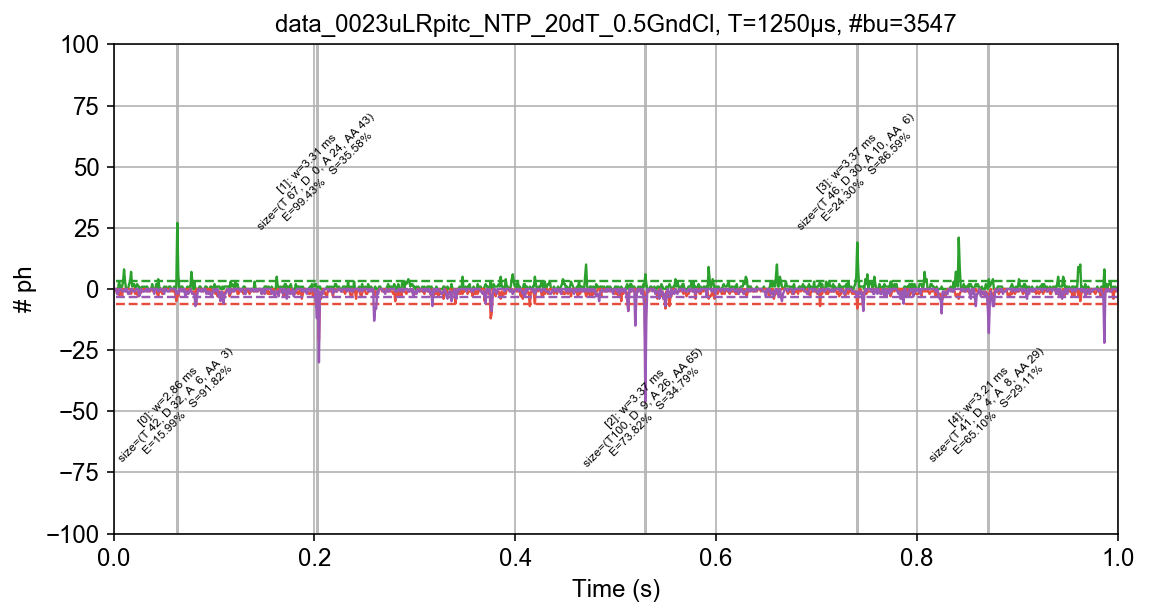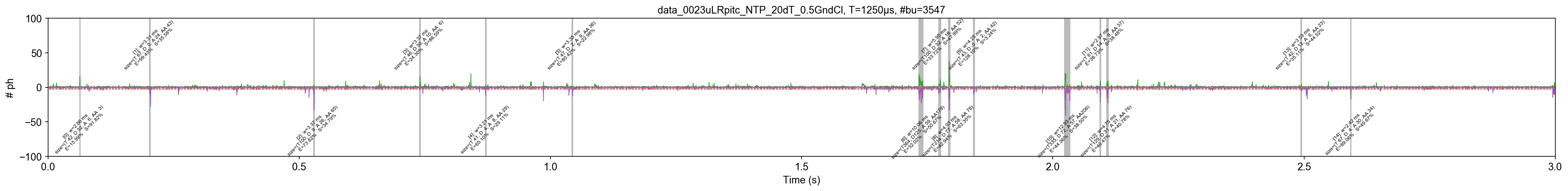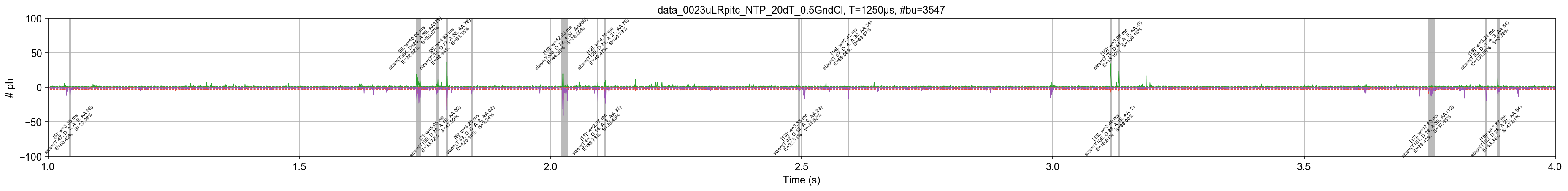# Example - Plotting timetraces with bursts¶

This notebook is part of smFRET burst analysis software FRETBursts.

In this notebook shows how to plot timetraces with burst information. For a complete tutorial on burst analysis see FRETBursts - us-ALEX smFRET burst analysis.

In :
from fretbursts import *

 - Optimized (cython) burst search loaded.
- Optimized (cython) photon counting loaded.
--------------------------------------------------------------
You are running FRETBursts (version 0.6.5+5.g5243bc2).

If you use this software please cite the following paper:

FRETBursts: An Open Source Toolkit for Analysis of Freely-Diffusing Single-Molecule FRET
Ingargiola et al. (2016). http://dx.doi.org/10.1371/journal.pone.0160716

--------------------------------------------------------------

In :
sns = init_notebook(apionly=True)
print('seaborn version: ', sns.__version__)

seaborn version:  0.8.0

In :
# Tweak here matplotlib style
import matplotlib as mpl
mpl.rcParams['font.sans-serif'].insert(0, 'Arial')
mpl.rcParams['font.size'] = 12
%config InlineBackend.figure_format = 'retina'
from IPython.display import display


# Get and process data¶

In :
url = 'http://files.figshare.com/2182601/0023uLRpitc_NTP_20dT_0.5GndCl.hdf5'
full_fname = "./data/0023uLRpitc_NTP_20dT_0.5GndCl.hdf5"

d.calc_bg(bg.exp_fit, time_s=1000, tail_min_us=(800, 4000, 1500, 1000, 3000))
d.burst_search(min_rate_cps=8e3)
dsf = ds.fuse_bursts(ms=10)

URL:  http://files.figshare.com/2182601/0023uLRpitc_NTP_20dT_0.5GndCl.hdf5
File: 0023uLRpitc_NTP_20dT_0.5GndCl.hdf5

# Total photons (after ALEX selection):     2,259,522
#  D  photons in D+A excitation periods:      721,537
#  A  photons in D+A excitation periods:    1,537,985
# D+A photons in  D  excitation period:     1,434,842
# D+A photons in  A  excitation period:       824,680

- Calculating BG rates ... [DONE]
- Performing burst search (verbose=False) ...[DONE]
- Calculating burst periods ...[DONE]
- Counting D and A ph and calculating FRET ...
- Applying background correction.
[DONE Counting D/A]
- - - - - CHANNEL  1 - - - -
--> END Fused 1186 bursts (25.1%, 9 iter)

- Counting D and A ph and calculating FRET ...
- Applying background correction.
[DONE Counting D/A and FRET]


# Plot Timetraces¶

Default plot:

In :
dplot(dsf, timetrace, tmin=0, tmax=1, bursts=True);We can plot a longer figure that scrolls horizontally in the notebook:

In :
fig, ax = plt.subplots(figsize=(32, 3))
dplot(dsf, timetrace, tmin=0, tmax=3, binwidth=0.5e-3, bursts=True,
ax=ax, plot_style=dict(lw=0.7))
plt.xlim(0, 3)
plt.grid(False)Using the previous plot we can sample different times of the measurement to have an overview of the timetrace:

In :
dx = dsf
num_time_points = 6
window = 3
kws = dict(figsize=(32, 3), bursts=True, binwidth=0.5e-3,
plot_style=dict(lw=0.7))

# Timepoints equally distributed along the measurement
time_points = np.round(np.linspace(dx.time_min+1, dx.time_max-window-1, num=num_time_points))

for i in time_points:
ax = dplot(dx, timetrace, tmin=i, tmax=i+window, **kws);
plt.xlim(i, i+window)
display(plt.gcf())
plt.close(plt.gcf())